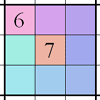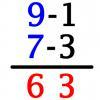# Search by Topic

#### Resources tagged with Multiplication & division similar to Sixty-seven Squared:

Filter by: Content type:
Age range:
Challenge level:

### There are 10 results

Broad Topics > Calculations and Numerical Methods > Multiplication & division### Sixty-seven Squared

##### Age 16 to 18 Challenge Level:

Evaluate these powers of 67. What do you notice? Can you convince someone what the answer would be to (a million sixes followed by a 7) squared?### What Is the Question?

##### Age 11 to 16 Challenge Level:

These pictures and answers leave the viewer with the problem "What is the Question". Can you give the question and how the answer follows?### Number Rules - OK

##### Age 14 to 16 Challenge Level:

Can you convince me of each of the following: If a square number is multiplied by a square number the product is ALWAYS a square number...### Expenses

##### Age 14 to 16 Challenge Level:

What is the largest number which, when divided into 1905, 2587, 3951, 7020 and 8725 in turn, leaves the same remainder each time?### More Mods

##### Age 14 to 16 Challenge Level:

What is the units digit for the number 123^(456) ?### Factorial

##### Age 14 to 16 Challenge Level:

How many zeros are there at the end of the number which is the product of first hundred positive integers?### Times Right

##### Age 11 to 16 Challenge Level:

Using the digits 1, 2, 3, 4, 5, 6, 7 and 8, mulitply a two two digit numbers are multiplied to give a four digit number, so that the expression is correct. How many different solutions can you find?### Integrated Product Sudoku

##### Age 11 to 16 Challenge Level:

This Sudoku puzzle can be solved with the help of small clue-numbers on the border lines between pairs of neighbouring squares of the grid.### Galley Division

##### Age 14 to 16 Challenge Level:

Can you explain how Galley Division works?### Vedic Sutra - All from 9 and Last from 10

##### Age 14 to 16 Challenge Level:

Vedic Sutra is one of many ancient Indian sutras which involves a cross subtraction method. Can you give a good explanation of WHY it works?